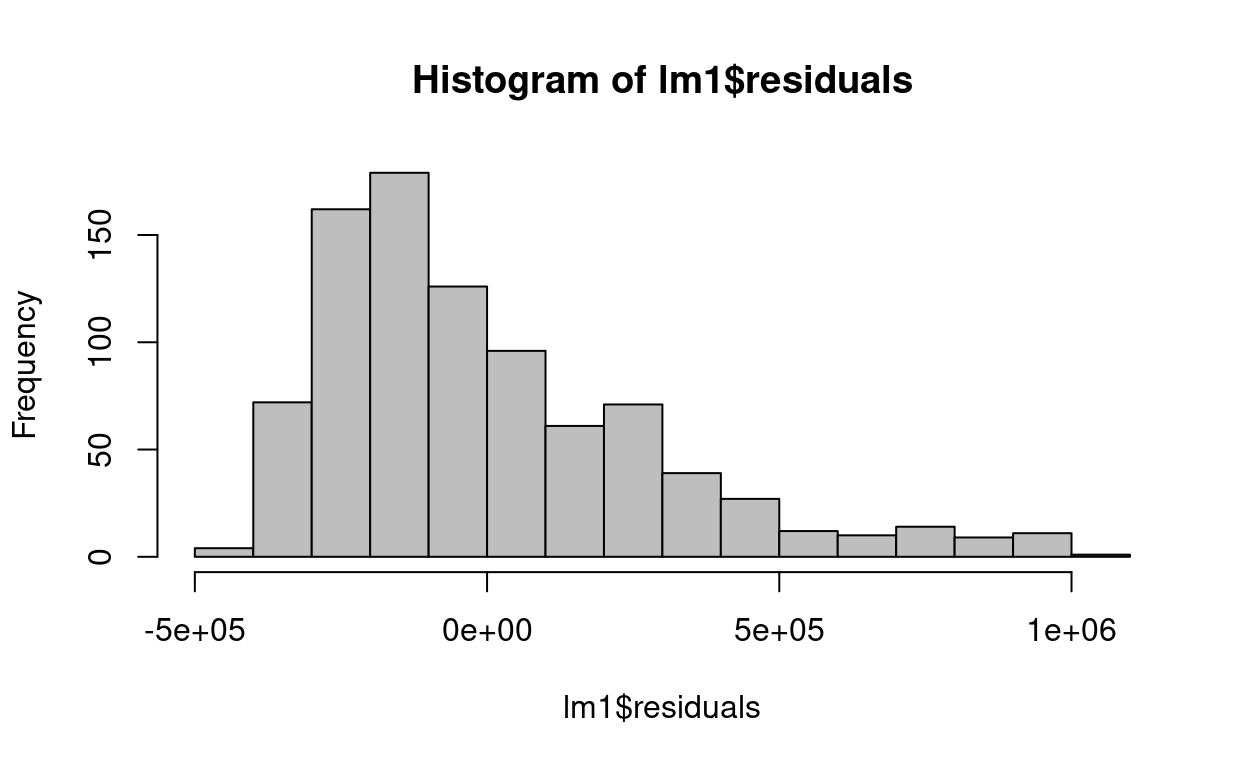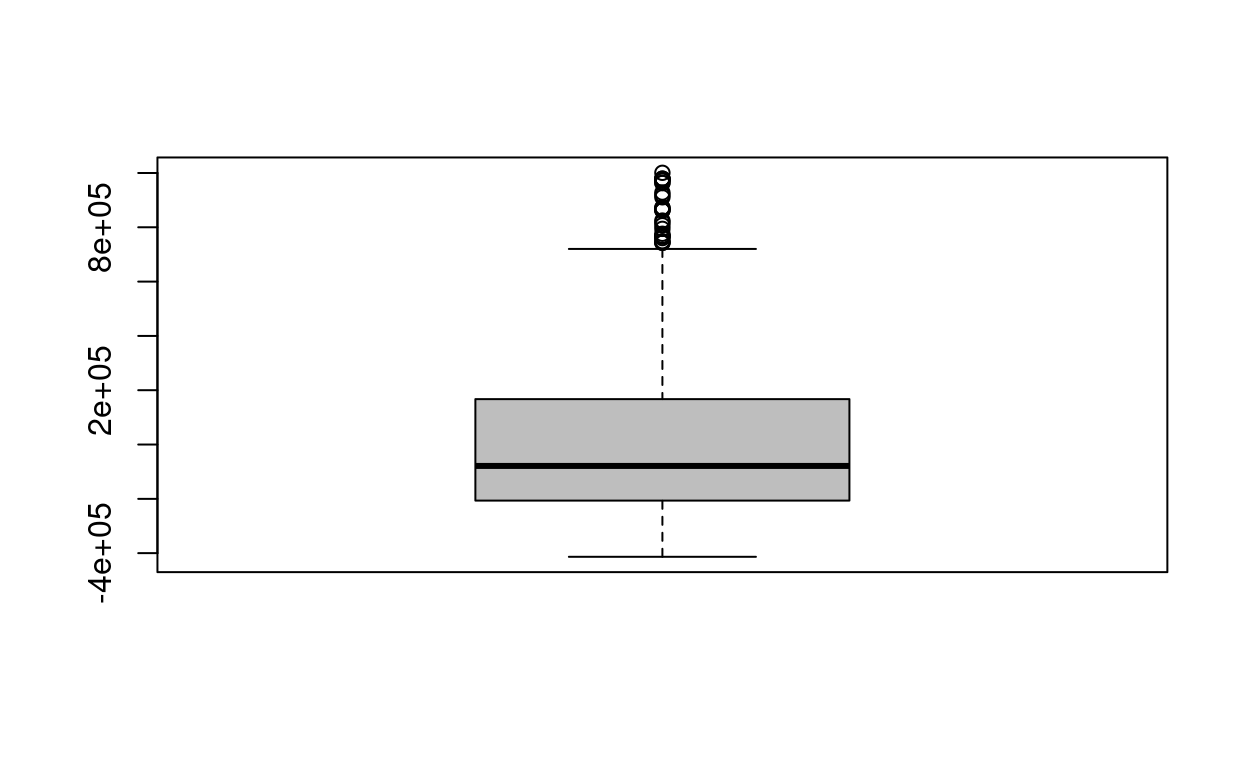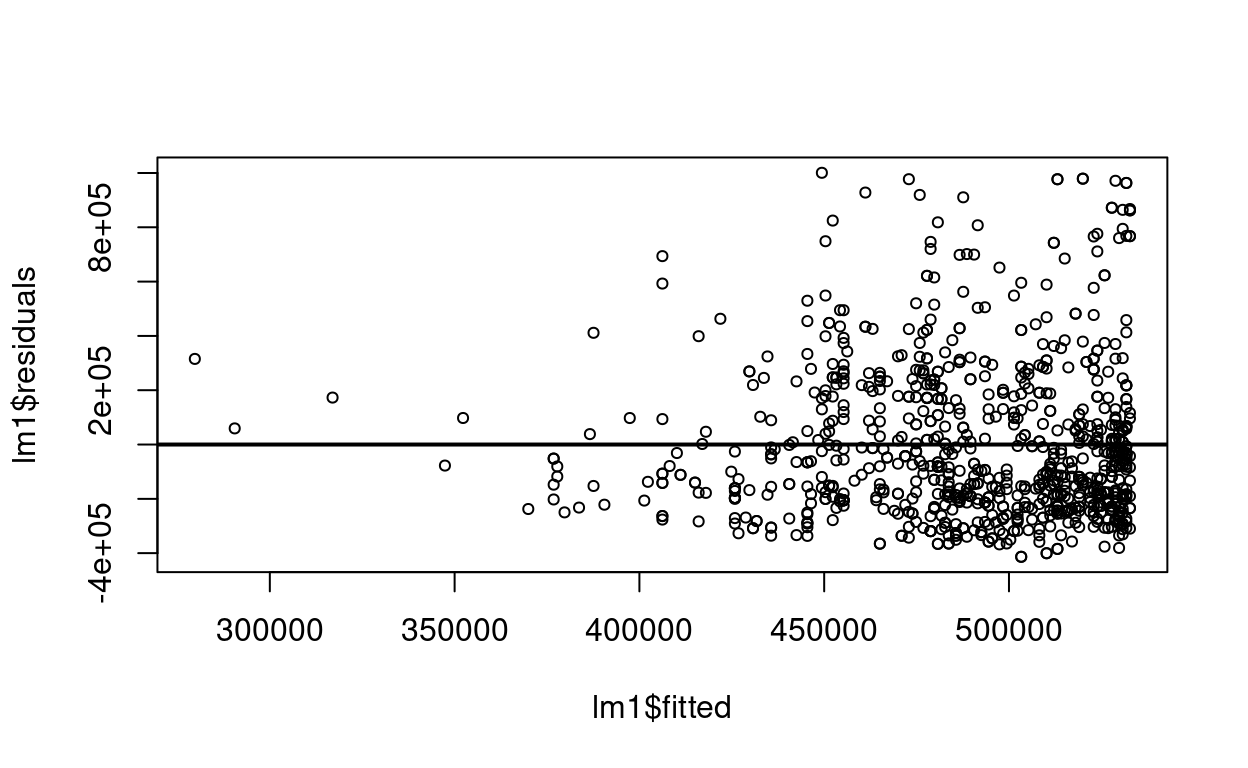A linear regression model was fit to predict how much money a customer would spend at an online retailer (in CAN$) based on the amount of time they were browsing the website (ranging between 1 and 100 minutes) along with the quadratic term for time. The coefficients table from the R output is: Estimate SE t-value Pr(>|t|) Intercept 6.902146 0.900384 7.666 9.41e-14 *** time 1.598175 0.122716 13.023 <2e-16 *** I(time^2) -0.008558 0.002852 -3.001 0.00282 ** ## Binary & categorical independent variables The main selling price of a sample of condos in Montreal was calculated to be$740,000 while the mean selling price of single family homes was calculated to the $975,000. If a regression mode was fit to predict selling price of a home based on a binary predictor for whether it was a condo ( x = 1 represents the condominium group). ## Interactions A regression model was fit to predict selling price of condos and single family homes in Montreal from $$x_1 = house~size$$, $$x_2 =$$ a binary independent variable for whether a home is a single family home ($$x_2 = 1$$ for single family homes) and the interaction between the two. the estimated regression model is given below: $\hat y = 428 + 0.286 x_1 + 104 x_2 - 0.140 (x_1 \times x_2)$ The regression model from the previous part is repeated here: to predict selling price of condominiums and single-family homes in Cambridge from x1 = house size, x2 = a binary predictor for whether a home is a single family home (x2 = 1 for single family homes), and the interaction between the two. The estimated regression model is given below: $\hat y = 428 + 0.286 x_1 + 104 x_2 - 0.140(x_1 \times x_2)$ ## Comparing models Two regression models are to be considered: Model 1: $$y = \beta_0 + \beta_1 x_1$$ Model 2: $$y = \beta_0 + \beta_1 x_1 + \beta_2 x_2 + \beta_3 x_3$$ Two regression models are to be considered: Model 1: $$y = \beta_0 + \beta_1 x_1$$ Model 2: $$y = \beta_0 + \beta_1 x_2 + \beta_2 x_3$$ ## Automatic Model Selection ## Model diagnostics You’d like to determine whether the normal distribution assumption is reasonable for a simple linear regression model. Let us fit a linear regression: re = read.csv("Real_Estate_Sample.csv") lm1 = lm(Price ~ year, data=re) The following histogram was produced after fitting the previous simple linear regression: re = read.csv("Real_Estate_Sample.csv") lm1 = lm(Price ~ year, data=re) hist(lm1$residuals,col="gray")boxplot(lm1$residuals,col="gray")The following residual-versus-predicted scatterplot was produced after fitting a simple linear regression of Y on X: plot(lm1$residuals~lm1\$fitted,cex=0.7)
abline(h=0,lwd=2)